IdeaScale Support Center

# Assessment Stage Rating Calculation

In Assessment stage, each idea undergoes a process where administrator request additional queries on the idea for evaluation. Evaluation may vary in number of evaluators or the number of questions to be asked. Participants evaluate the ideas based assessment questions on a scale of 1 to 5 stars.

The rating is calculated on the following methodology:

Scenario:

1. One idea has 2 (two) assessment questions and each question has been rated as 5 stars
2. Number of participants 3 (three) for assessing the idea

Assessment Rating Calculation:

1. One participant rated both the question as 5 stars, so the rating calculation will be 5 star + 5 star = 10 (Individual Idea Rating)  and 10/2 = 5  (Idea Rating)

( "2" is the no of assessment question)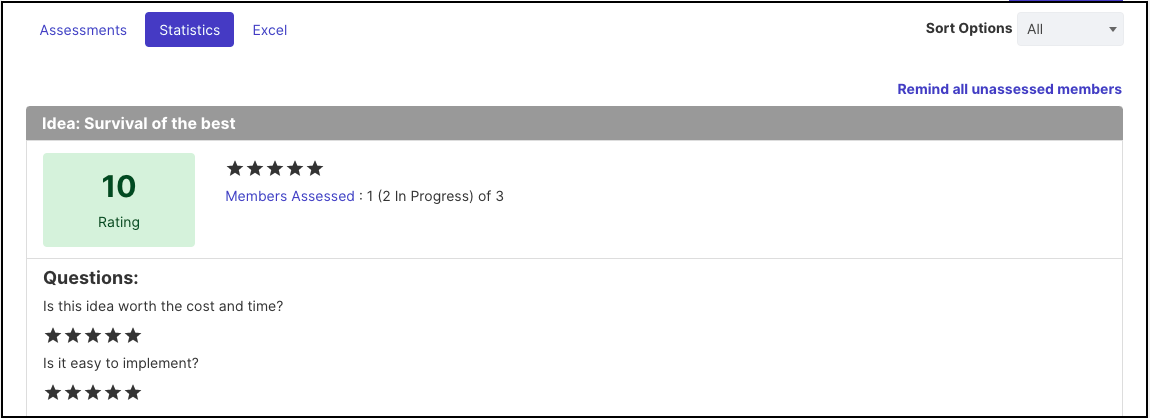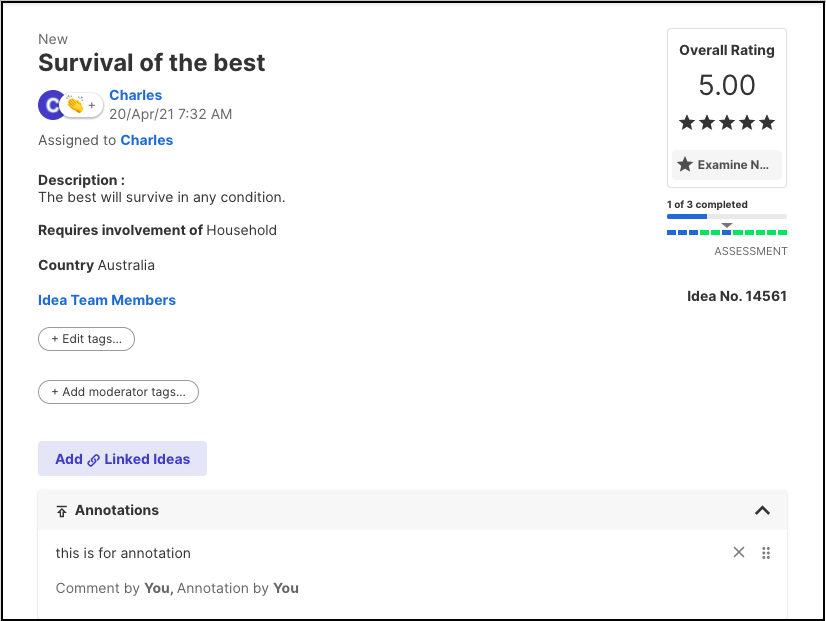2. Another member rated one question as 3 star and other question as 2 star, so the rating calculation will be
3 star + 2 star = 5 (Individual Idea Rating) and 5 /2= 2.5 (Idea Rating)
( "2" is the no of assessment question)

Total idea rating (individual): 5 star + 2.5 star = 7.5

And The overall idea rating is, 7.5/2 = 3.75
(Note: Here 2 is the number of participants who have assessed)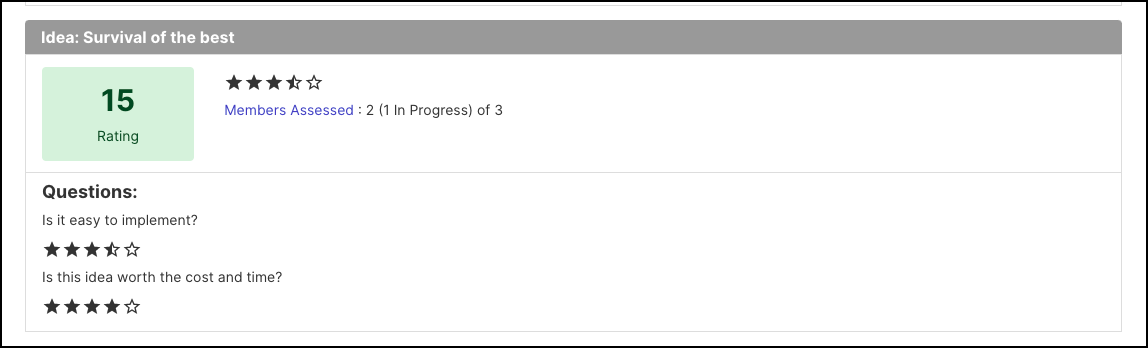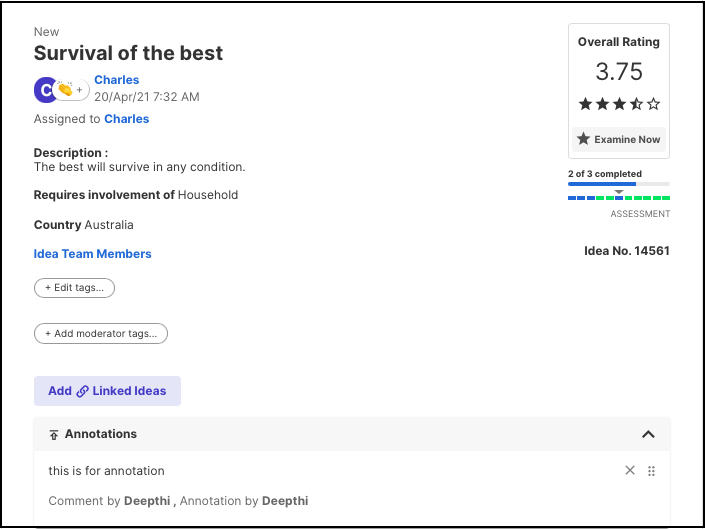When NA is selected as an answer then that criteria is removed from the equation. It does not factor in the score.

The total no of star given by the participants can be viewed from Assessment statistics. Statistics tab displays the total star given by each participant for each idea.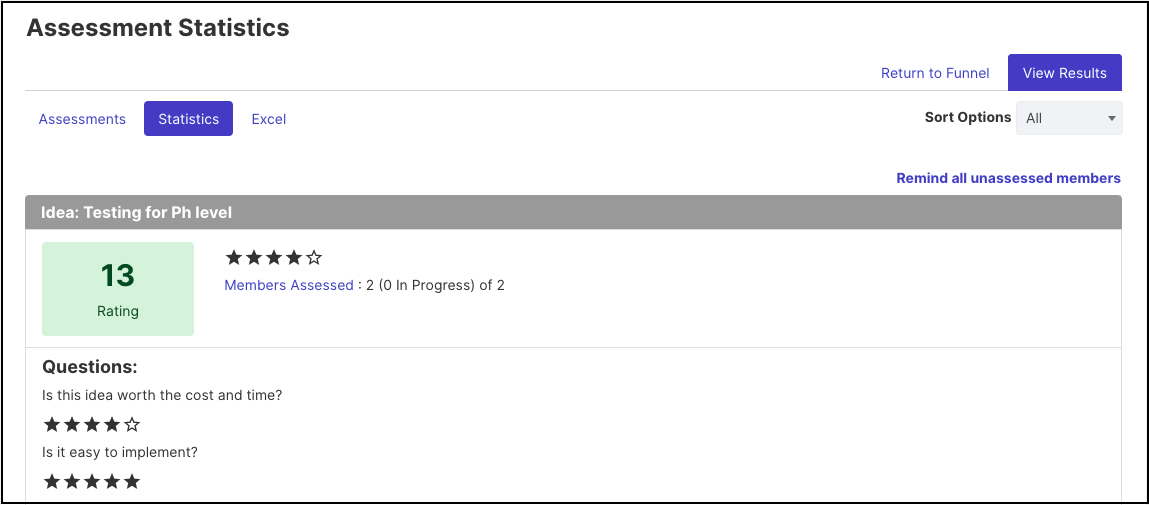Last Updated: July 5, 2023